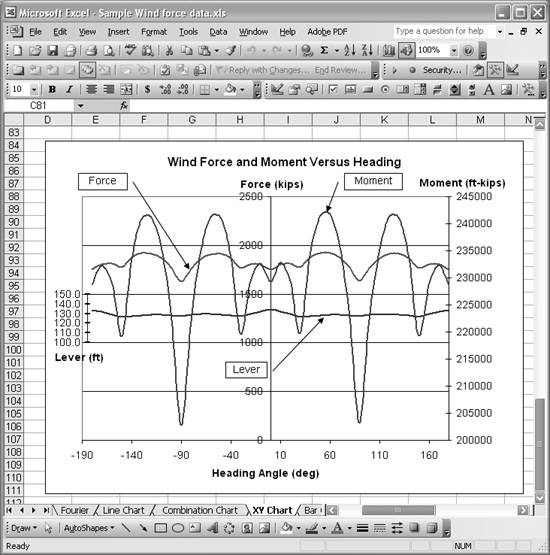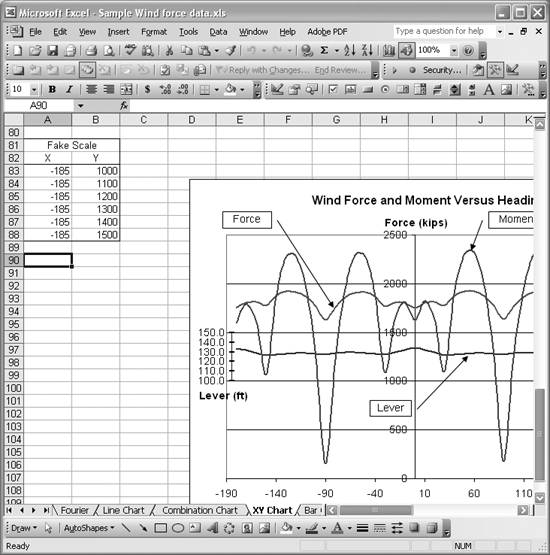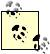# Using Multiple Axes

Problem

You've plotted several series on a chart, but one of them consists of values much larger than the others; when it is plotted on the same scale, you can barely discern the smaller values. Thus, you'd like to plot multiple data series on a single chart but with different scales.

Solution

You can use multiple scales. Excel has built-in support for secondary axes . This allows you to plot, for example, one data series using a primary y-axis and another data series using a secondary y-axis. To specify the axis for a data series, select the series and then select Format [Image_Link]/images/U2192.jpg border=0> Selected Data Series...from the main menu bar to open the Format Data Series dialog box . Click the Axis tab and then click either "Primary axis" or "Secondary axis" to specify the axis. Press OK when you're done, and Excel will automatically set up another axis and rescale your series.

Discussion

Figure 4-14 shows an example of two data series with very different scales plotted on the same chart.

Figure 4-14. Two data series with very different scalesThe scales of these two sets of data are so different that when they are plotted on the same chart, you can barely even see one of the data series; it's almost coincident with the x-axis.

Changing the axis of this series so that it's plotted on a secondary y-axis (as described earlier) results in the chart shown in Figure 4-15.

Figure 4-15. Chart using a secondary y-axisThis version of the chart is far more readable than the original, and shows how useful secondary axes are. You can adjust the position of each series relative to the other by changing the minimum and maximum values of the primary or secondary axes.

You can, of course, plot more than one series relative to either the primary or secondary axes. Unfortunately, Excel does not support more than one secondary axis. While this isn't the end of the world, there are times when more than one secondary axis would be useful. In these cases, there are a couple of workarounds you can try.

The easiest thing to try is to scale your data. For example, multiply (or divide) a data series by 10, 100, 1,000, or some other appropriate value and then plot it against the primary or secondary axis. Be sure to label the series properly so readers of your chart can clearly see that the data is scaled.

Another trick you might find useful is to fake another axis by using a specifically crafted dummy series. Let's say we wanted to plot another series on the chart shown in Figure 4-15, but the values of this new series ranged from about 100 to 150. Clearly such a series would get buried by the scale of values for the other two series.

The solution is to multiply the values in this new series by an appropriate scale factor, say 10, and then plot it against the primary axis. This would make the values range from about 1,000 to 1,500, which would be clearly visible on the chart. Confusion may arise, however, if the scale is not annotated to indicate the new series has been scaled. To get around this, you can create a fake scale using a dummy series. Figure 4-16 shows just such a fake scale.

Figure 4-16. Fake scaleThe series labeled Lever is the new, scaled series plotted against the primary y-axis (the center one). The fake axis is the short axis to the left, labeled Lever. This technique allows all of these series to be plotted on the same chart, even though the series values vary widely in magnitude.

To create the fake axis, you need to create and add a dummy series. The dummy series I created for this example is shown in Figure 4-17.

Figure 4-17. Fake series dataThe key to using a series to represent an axis is to make one of the coordinates constant. In this case, since I want the series to represent a vertical axis, I set the x-value for the series to a constant. I used -185 to position the series all the way to the left of the chart. The y-values are simply selected to capture an appropriate range of values. Once the series data is prepared, you can add the series to the chart as discussed in Recipe 4.1. You'll also want to turn on Y-labels for the dummy series. You can turn on Y-labels by opening the Format Data Series dialog box and selecting the Data Labels tab. Upon doing so, you'll notice a little hiccup that you have to correct.

The default Y-Labels for the dummy series displays the actual y-values. The problem is that these values represent the scaled values for the series we wanted to use the fake axis for in the first place. This isn't what we want, so you must edit each data label manually to display the appropriate values. Click the series labels once to select all of the labels. Then click once again on the specific label you want to modify. This will put the label in edit mode, where you can change the label to whatever you desire. Make the changes to all of the labels and you'll end up with something like the chart shown in Figure 4-16.You can use this fake axis technique to add any number of fake horizontal or vertical axes.Excel Scientific and Engineering Cookbook (Cookbooks (OReilly))
ISBN: 0596008791
EAN: 2147483647
Year: N/A
Pages: 206
Authors: David M Bourg